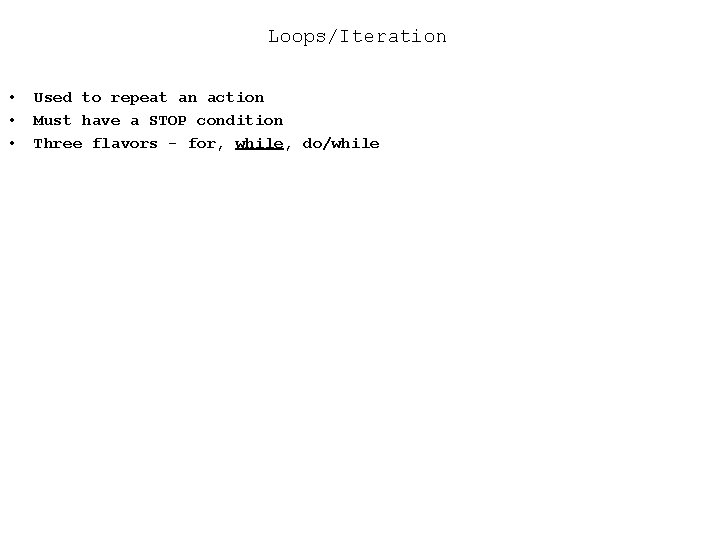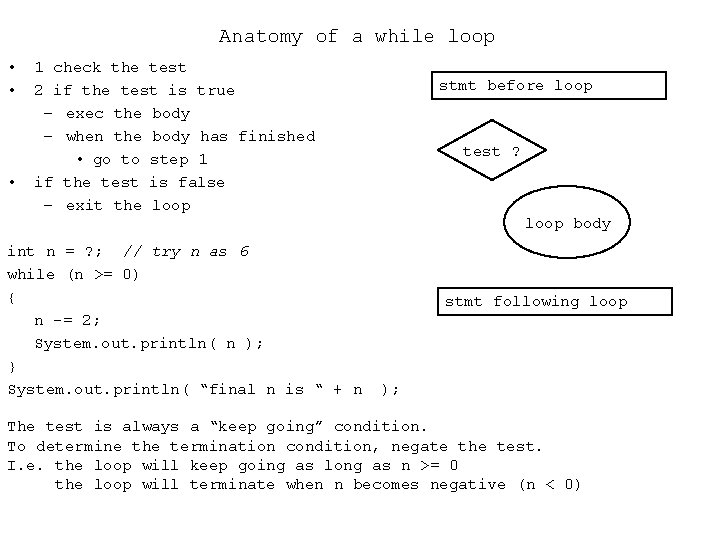# LoopsIteration Used to repeat an action Must have

• Slides: 6Loops/Iteration • • • Used to repeat an action Must have a STOP condition Three flavors - for, while, do/whileAnatomy of a while loop • • • 1 check the test 2 if the test is true – exec the body – when the body has finished • go to step 1 if the test is false – exit the loop int n = ? ; // try n as 6 while (n >= 0) { n -= 2; System. out. println( n ); } System. out. println( “final n is “ + n stmt before loop test ? loop body stmt following loop ); The test is always a “keep going” condition. To determine the termination condition, negate the test. I. e. the loop will keep going as long as n >= 0 the loop will terminate when n becomes negative (n < 0)while Loops • • The test is checked at the very beginning and then again each time after the entire loop body has been executed The test is NOT checked in the middle of the loop body This is true for all the loops (for, while, and do/while), not just the while loop A while is just an if statement that keeps going back to the test and quits looping at first failure of test x = ? ; // try x as 45 while (x < 50) { x++; System. out. println( x ); }Practice What’s the output? int d = 90; while (d < 80) { ++d; } System. out. println( “d is “ + d ); int x = 90; while (x < 100) { x += 5; if (x>95) x-=25; } System. out. println(“final value for x is “ + x ); int z = 85; while (z < 90) { z -= 5; } System. out. println(“final value for z is “ + z );Summing (even) numbers with a while loop Example of an indeterminate loop - the user’s input will determine how many times the loop executes // assume kbd declared (save space) int sum = 0, evensum = 0, number; System. out. print(“ First number please “); number = Integer. parse. Int( kbd. read. Line() ); while (number > 0) { sum += number; if (number % 2 == 0) { evensum += number; } System. out. print(“number please “); number = Integer. parse. Int( kbd. read. Line() ); } System. out. println(“sum is “ + sum ); System. out. println(“sum of even #’s is “ + evensum );Error checking with a do loop is a variant of the while that waits till the bottom to make the test. There are some very good uses for the do form of the while final int MAX = 10, MIN = 5; int number; do { System. out. print(“Enter # between “ + “ 5 and 10 inclusive: “); number = Integer. parse. Int( kbd. readl. Line() ); if (number < MIN || number > MAX) System. out. println(“: =( Try again”); } while (number < MIN || number > MAX); System. out. print(“The user entered “ + number ); stmt before loop test ? loop body stmt following loop